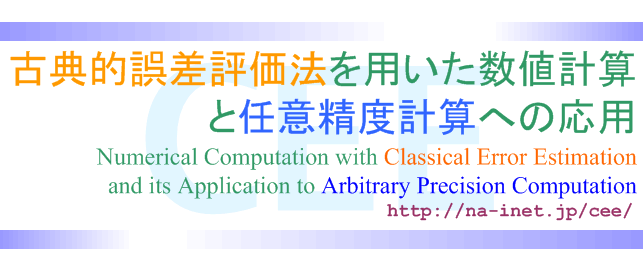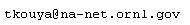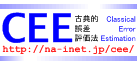Japanese Page

Last Update: 2008-07-01

• "Classical Error Estimation (CEE)" is :
• a posteriori and empirical error estimation which has been used for long time
• not regorous but practical one because it does not require to rewrite existed numerical algorithmes
• to separate round-off error in approximation obtained, so truncation error (theoritical error) in it can be estimated.
• applicable in IEEE754 double (and fixed) precision) ONLY
• able to be more accurate if multiple (and mixed) precision arithmetic would be available
• able to make us calculate the approximation with user-required precision as application of CEE
• How do we apply CEE to arbitrary precision computation ?
• Estimation of Round-off Error
• IEEE754 double and fixed precision arithmetic ONLY
• Multiple and mixed precision arithmetic like MPFR/GMP
• Case Study